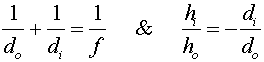Formula review

The following two formulas apply to both mirrors and lenses:The greatest difficulty is in remembering the signs of the variables. I think the easiest way to do it is the following:

1. do is always positive
2. di is positive for a real image, which is of course in front of a mirror, or behind a lens.
3. f is positive for a converging lens or mirror.

All of the possibilities are listed in the following table. I think this is an unnecessarily complicated way of summarizing the different cases; but some students prefer to have it stated this way:

 focal length object distance image distance concave mirror positive positive positive, if on the same side as object (real) negative, if on the opposite side as object (virtual) convex mirror negative positive negative,as all images are virtual and on the opposite side as object (virtual) converging lens positive positive positive, if on the opposite side as object (real) negative, if on the same side as object (virtual) diverging lens negative positive negative,as all images are virtual and on the same side as object (virtual)

Truths for both lenses and mirrors

1. Image distances are always negative for virtual images and positive for real images.

2. Object distances are always positive.

3. Real images are always inverted and virtual images are upright.

Lenses index      examples        Lecture index